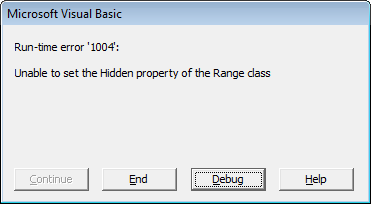﻿ Hide a row using VBA in Excel

# Hide rows using VBA in Excel

## Hiding one or more rows on a worksheet

Hiding a row can be simple. Below code hides row C on the active worksheet. If you set the property `Hidden` to `False` the row becomes visible again.

``Rows(2).Hidden = True``

Below code hides multiple rows (2, 3 and 4).

``Rows("2:4").Hidden = True``

## Hiding rows in a rangeWhen starting with a range which is not a complete row you will get run-time error '1004': Unable to set the Hidden property of the Range class.

``````Dim rng As Range: Set rng = Application.Range("B2")
rng.Hidden = True``````

You can fix this by applying EntireRow to the range:

``````Dim rng As Range: Set rng = Application.Range("B2")
rng.EntireRow.Hidden = True``````

## Make a row xlVeryHidden

xlVeryHidden is not supported for rows. A workaround is to hide it and then protect the sheet.